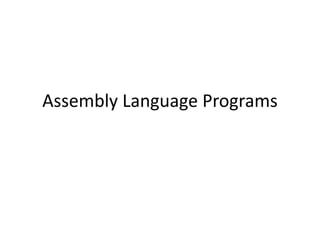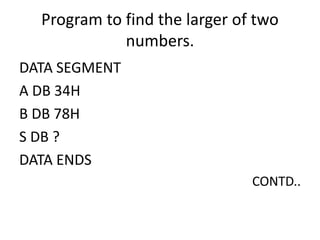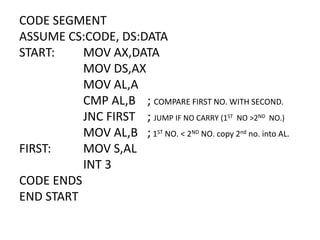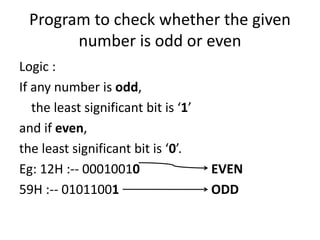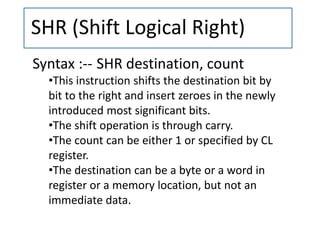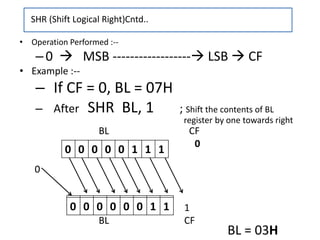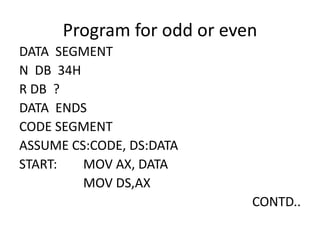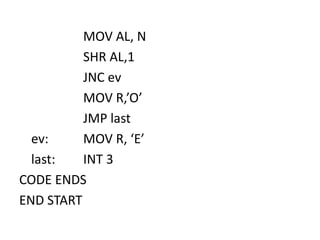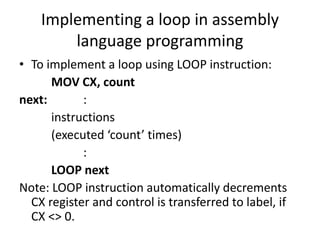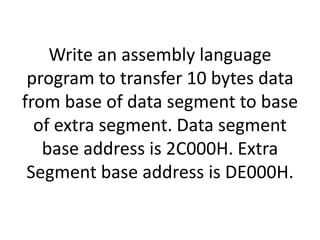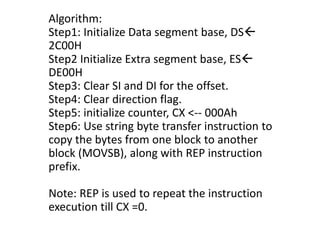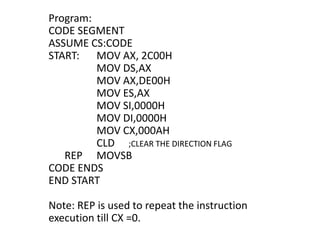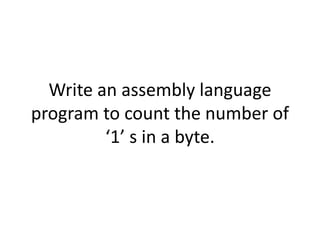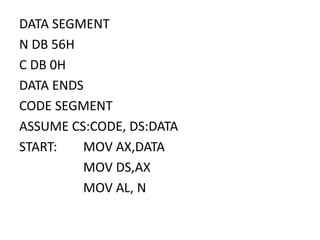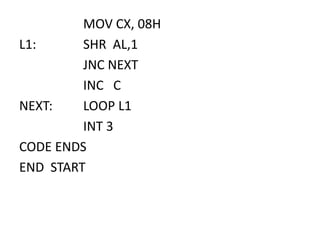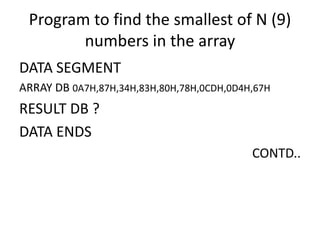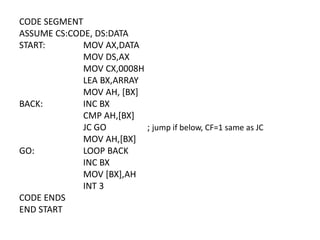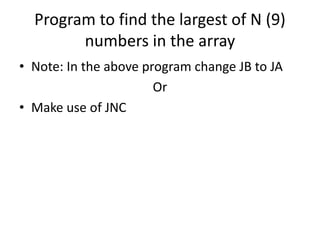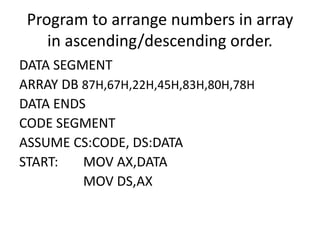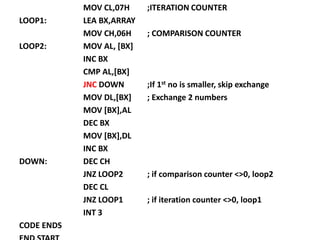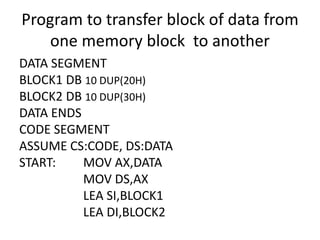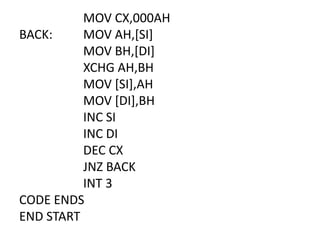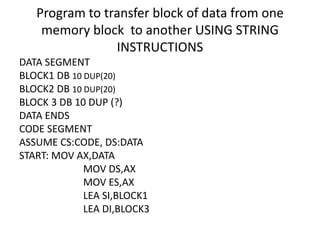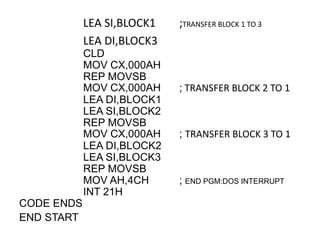1 de 24

### Assembly language programs

1. Assembly Language Programs
2. Program to find the larger of two numbers. DATA SEGMENT A DB 34H B DB 78H S DB ? DATA ENDS CONTD..
3. CODE SEGMENT ASSUME CS:CODE, DS:DATA START: MOV AX,DATA MOV DS,AX MOV AL,A CMP AL,B ; COMPARE FIRST NO. WITH SECOND. JNC FIRST ; JUMP IF NO CARRY (1ST NO >2ND NO.) MOV AL,B ;1ST NO. < 2ND NO. copy 2nd no. into AL. FIRST: MOV S,AL INT 3 CODE ENDS END START
4. Program to check whether the given number is odd or even Logic : If any number is odd, the least significant bit is ‘1’ and if even, the least significant bit is ‘0’. Eg: 12H :-- 00010010 EVEN 59H :-- 01011001 ODD
5. SHR (Shift Logical Right) Syntax :-- SHR destination, count •This instruction shifts the destination bit by bit to the right and insert zeroes in the newly introduced most significant bits. •The shift operation is through carry. •The count can be either 1 or specified by CL register. •The destination can be a byte or a word in register or a memory location, but not an immediate data.
6. BL CF 0 0 1 BL CF 0 0 0 0 0 0 1 1 • Operation Performed :-- –0  MSB ------------------ LSB  CF • Example :-- – If CF = 0, BL = 07H – After SHR BL, 1 ; Shift the contents of BL register by one towards right SHR (Shift Logical Right)Cntd.. 0 0 0 0 0 1 1 1 BL = 03H
7. Program for odd or even DATA SEGMENT N DB 34H R DB ? DATA ENDS CODE SEGMENT ASSUME CS:CODE, DS:DATA START: MOV AX, DATA MOV DS,AX CONTD..
8. MOV AL, N SHR AL,1 JNC ev MOV R,’O’ JMP last ev: MOV R, ‘E’ last: INT 3 CODE ENDS END START
9. Implementing a loop in assembly language programming • To implement a loop using LOOP instruction: MOV CX, count next: : instructions (executed ‘count’ times) : LOOP next Note: LOOP instruction automatically decrements CX register and control is transferred to label, if CX <> 0.
10. Write an assembly language program to transfer 10 bytes data from base of data segment to base of extra segment. Data segment base address is 2C000H. Extra Segment base address is DE000H.
11. Algorithm: Step1: Initialize Data segment base, DS 2C00H Step2 Initialize Extra segment base, ES DE00H Step3: Clear SI and DI for the offset. Step4: Clear direction flag. Step5: initialize counter, CX <-- 000Ah Step6: Use string byte transfer instruction to copy the bytes from one block to another block (MOVSB), along with REP instruction prefix. Note: REP is used to repeat the instruction execution till CX =0.
12. Program: CODE SEGMENT ASSUME CS:CODE START: MOV AX, 2C00H MOV DS,AX MOV AX,DE00H MOV ES,AX MOV SI,0000H MOV DI,0000H MOV CX,000AH CLD ;CLEAR THE DIRECTION FLAG REP MOVSB CODE ENDS END START Note: REP is used to repeat the instruction execution till CX =0.
13. Write an assembly language program to count the number of ‘1’ s in a byte.
14. DATA SEGMENT N DB 56H C DB 0H DATA ENDS CODE SEGMENT ASSUME CS:CODE, DS:DATA START: MOV AX,DATA MOV DS,AX MOV AL, N
15. MOV CX, 08H L1: SHR AL,1 JNC NEXT INC C NEXT: LOOP L1 INT 3 CODE ENDS END START
16. Program to find the smallest of N (9) numbers in the array DATA SEGMENT ARRAY DB 0A7H,87H,34H,83H,80H,78H,0CDH,0D4H,67H RESULT DB ? DATA ENDS CONTD..
17. CODE SEGMENT ASSUME CS:CODE, DS:DATA START: MOV AX,DATA MOV DS,AX MOV CX,0008H LEA BX,ARRAY MOV AH, [BX] BACK: INC BX CMP AH,[BX] JC GO ; jump if below, CF=1 same as JC MOV AH,[BX] GO: LOOP BACK INC BX MOV [BX],AH INT 3 CODE ENDS END START
18. Program to find the largest of N (9) numbers in the array • Note: In the above program change JB to JA Or • Make use of JNC
19. Program to arrange numbers in array in ascending/descending order. DATA SEGMENT ARRAY DB 87H,67H,22H,45H,83H,80H,78H DATA ENDS CODE SEGMENT ASSUME CS:CODE, DS:DATA START: MOV AX,DATA MOV DS,AX
20. MOV CL,07H ;ITERATION COUNTER LOOP1: LEA BX,ARRAY MOV CH,06H ; COMPARISON COUNTER LOOP2: MOV AL, [BX] INC BX CMP AL,[BX] JNC DOWN ;If 1st no is smaller, skip exchange MOV DL,[BX] ; Exchange 2 numbers MOV [BX],AL DEC BX MOV [BX],DL INC BX DOWN: DEC CH JNZ LOOP2 ; if comparison counter <>0, loop2 DEC CL JNZ LOOP1 ; if iteration counter <>0, loop1 INT 3 CODE ENDS
21. Program to transfer block of data from one memory block to another DATA SEGMENT BLOCK1 DB 10 DUP(20H) BLOCK2 DB 10 DUP(30H) DATA ENDS CODE SEGMENT ASSUME CS:CODE, DS:DATA START: MOV AX,DATA MOV DS,AX LEA SI,BLOCK1 LEA DI,BLOCK2
22. MOV CX,000AH BACK: MOV AH,[SI] MOV BH,[DI] XCHG AH,BH MOV [SI],AH MOV [DI],BH INC SI INC DI DEC CX JNZ BACK INT 3 CODE ENDS END START
23. Program to transfer block of data from one memory block to another USING STRING INSTRUCTIONS DATA SEGMENT BLOCK1 DB 10 DUP(20) BLOCK2 DB 10 DUP(20) BLOCK 3 DB 10 DUP (?) DATA ENDS CODE SEGMENT ASSUME CS:CODE, DS:DATA START: MOV AX,DATA MOV DS,AX MOV ES,AX LEA SI,BLOCK1 LEA DI,BLOCK3
24. LEA SI,BLOCK1 ;TRANSFER BLOCK 1 TO 3 LEA DI,BLOCK3 CLD MOV CX,000AH REP MOVSB MOV CX,000AH ; TRANSFER BLOCK 2 TO 1 LEA DI,BLOCK1 LEA SI,BLOCK2 REP MOVSB MOV CX,000AH ; TRANSFER BLOCK 3 TO 1 LEA DI,BLOCK2 LEA SI,BLOCK3 REP MOVSB MOV AH,4CH ; END PGM:DOS INTERRUPT INT 21H CODE ENDS END START